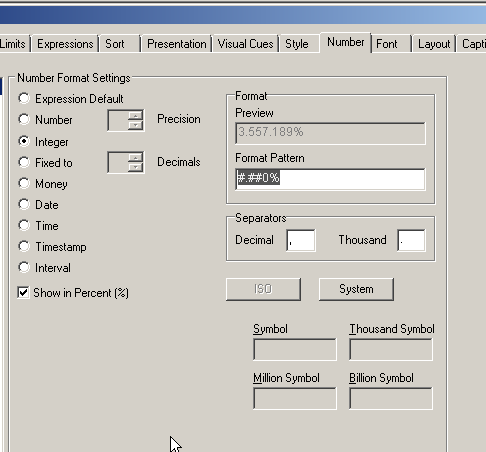# QlikView App Dev

Discussion Board for collaboration related to QlikView App Development.

Announcements
Coming Aug. 9: New Simplified Authoring for Qlik Sense SaaS – For Details, CLICK HERE
cancel
Showing results for
Did you mean:Not applicable

## Turning a formula into a % in a text box

Hi,

I have various text boxes on my dashboard with calculations  in them. I have some that are percentages but i cant format them correctly.

This is an example:

left(1-(sum({\$<Metric_Part={'Num'}, Metric_Code={'RTT24'}>} Metric_Value)
/
sum({\$<Metric_Part={'Den'}, Metric_Code={'RTT24'}>} Metric_Value ) )

,4 ) & '%'

Rather than 'left' what can i sue to be more accurate, i was thinking a 'round' function? and also the above calculation brings out 0.76%, when i need it to say 76%.

Thanks,

1 Solution

Accepted SolutionsLuminary Alumni

Hi,

Format the numbers using Num() like below

=Num(1-(sum({\$<Metric_Part={'Num'}, Metric_Code={'RTT24'}>} Metric_Value)
/
sum({\$<Metric_Part={'Den'}, Metric_Code={'RTT24'}>} Metric_Value ) ), '#,##0.0%')

Hope this helps you.

Regards,

Jagan.

7 RepliesMVP & Luminary

You could use something like num(value, '#.##0,0%').

- MarcusNot applicable
Author

Sorry - i dont understand. Where do i put that in my formula?MVP & Luminary

num(1-(sum({\$<Metric_Part={'Num'}, Metric_Code={'RTT24'}>} Metric_Value)
/
sum({\$<Metric_Part={'Den'}, Metric_Code={'RTT24'}>} Metric_Value ) ) , '#.##0,0%')MVP

If your expression (I'll call exp) is

1- (

sum({\$<Metric_Part={'Num'}, Metric_Code={'RTT24'}>} Metric_Value)  /

sum({\$<Metric_Part={'Den'}, Metric_Code={'RTT24'}>} Metric_Value )

)

To format your expression use num function, the format could be #.##0%

num(exp, '#.##0%')

To get the right format (bold), open the number tab in a chart, try the different format and when it's correct copy the format patternLuminary Alumni

Hi,

Format the numbers using Num() like below

=Num(1-(sum({\$<Metric_Part={'Num'}, Metric_Code={'RTT24'}>} Metric_Value)
/
sum({\$<Metric_Part={'Den'}, Metric_Code={'RTT24'}>} Metric_Value ) ), '#,##0.0%')

Hope this helps you.

Regards,

Jagan.Not applicable
Author

Thanks - that has workedLuminary Alumni

Hi,

If you got the answer please close this thread by giving correct and helpful answers to the useful posts, so that it would help others in finding the answers for similar type of questions.

Regards,

Jagan.